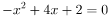# Simultaneous Equations - Graphical Representation

Topic:
Equations

## Simultaneous Equations involving a variables of degree 2

First solve these equations algebraically and . After find the solutions for x and y, check show function to show the graphs, then check show intersection to show the (x,y). In others the solution of simultaneous equations is / are the points of intersections of graphs in this case a parabola and a straight line.

## Investigation

Change the value 'm' and 'c' to 0. Observe what are the intersection points. Jot down the values. Do you find anything interseting ? or what these values are called with respect to a function or an equation?

## Investigation #2

Let us continue with 'm'= 0 and change 'c'. Thus the straight line will be parallel to ? quadratic equation continue to bemove 'c' to -1, -2 , - 3. jot down straight line equation and the corresponding points of intersection. you can further go up i.e. by moving 'c' to positive values. Again jot down the points of intersection.

## Investigation # 3

Continue with 'm' = 0 and play around with 'c' only. For what values of 'c' such the x-values are always positive? What happens when c = 6?

## Investigation # 4

What happens when c= 7 ? How can you generalise?

## Exam Question

For what values 'c' of and such that f(x) and g(x) do not intersect in a real Cartesian plane?